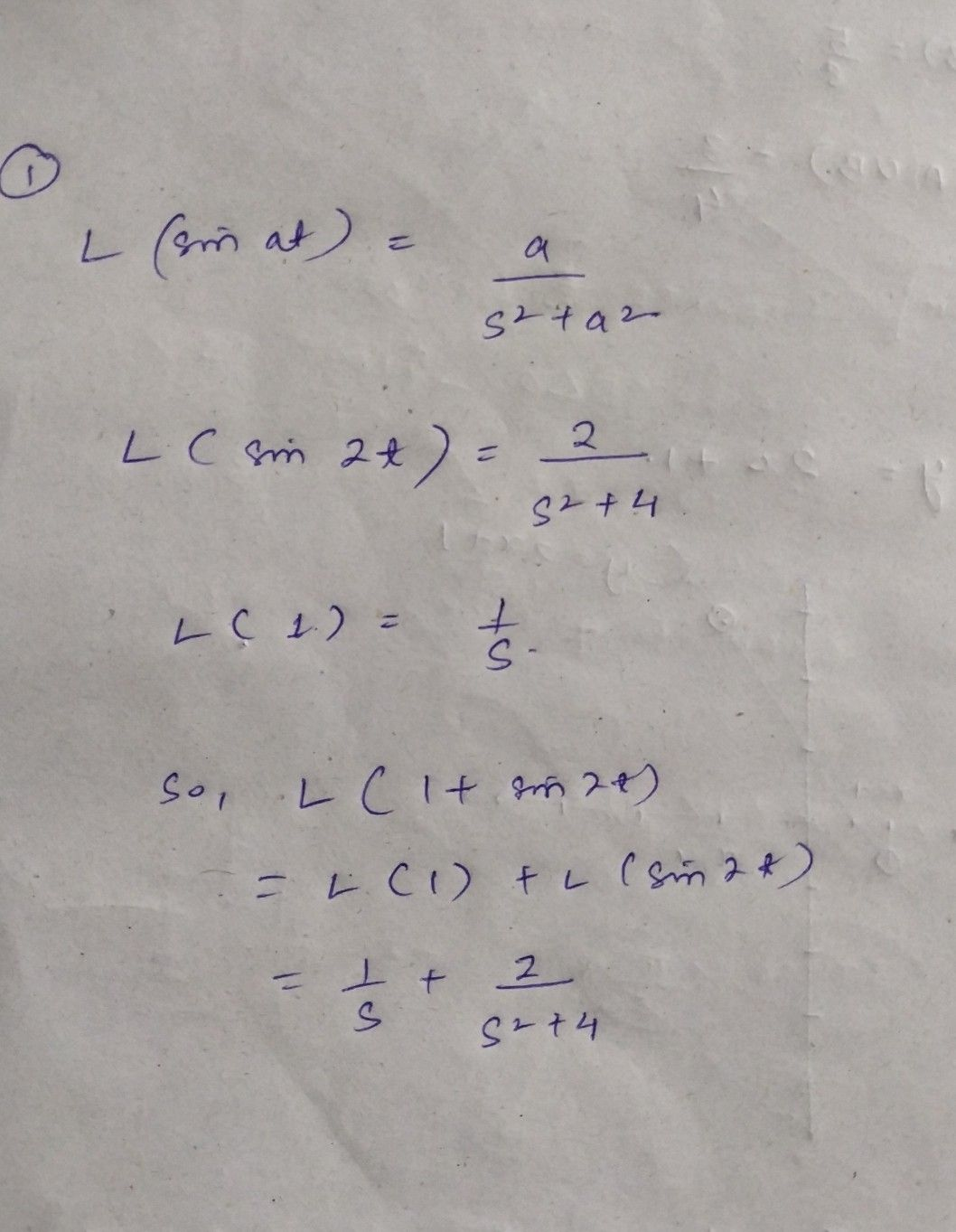Symbol
Problem$1$ Find the Laplace transform $f\left(1+sin2t\right)$ $2$ Find the Laplace transform wof ww sin at and cos $at$ $3$ Find the Laplace transform of of the functionf $t-1,$ $f\left(t\right)=\left(3-t$ $1<t<$ $2<t<$ $4$ IfL{f(t)} $\left(f\left(t\right)\right)=F\left(s\right),$ then to prove that $1$ $s$ $\left(f\left(at\right)\right)=-F\left(-\right)$
Other
Search count: 124
SolutionQanda teacher - SambitStudent
4th ques solution I wantQanda teacher - Sambit
I don't remember the proofStudent
ooho no problem